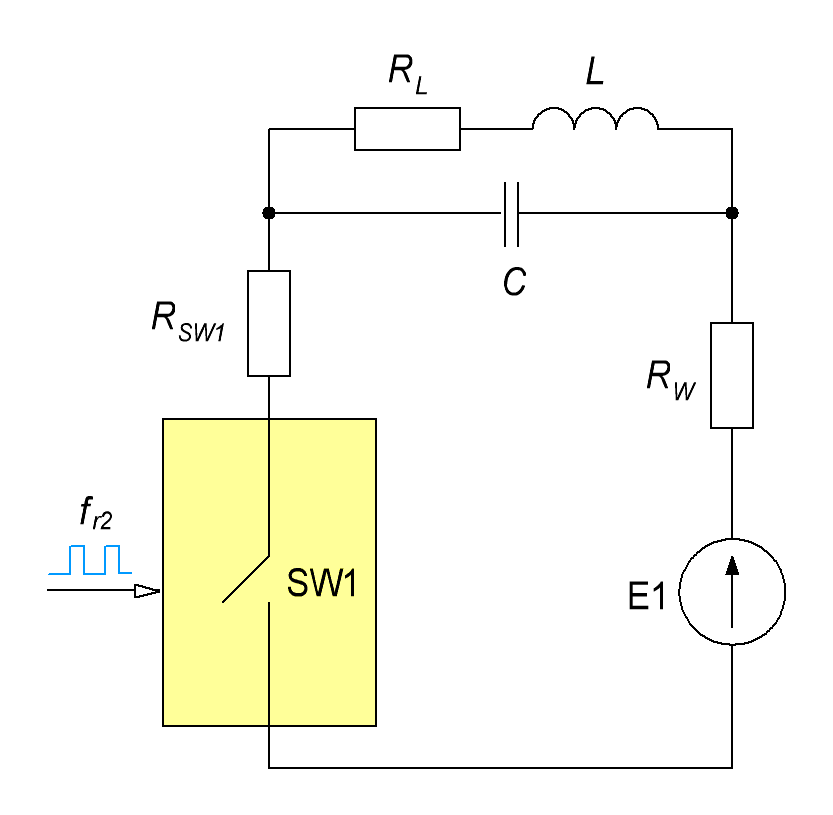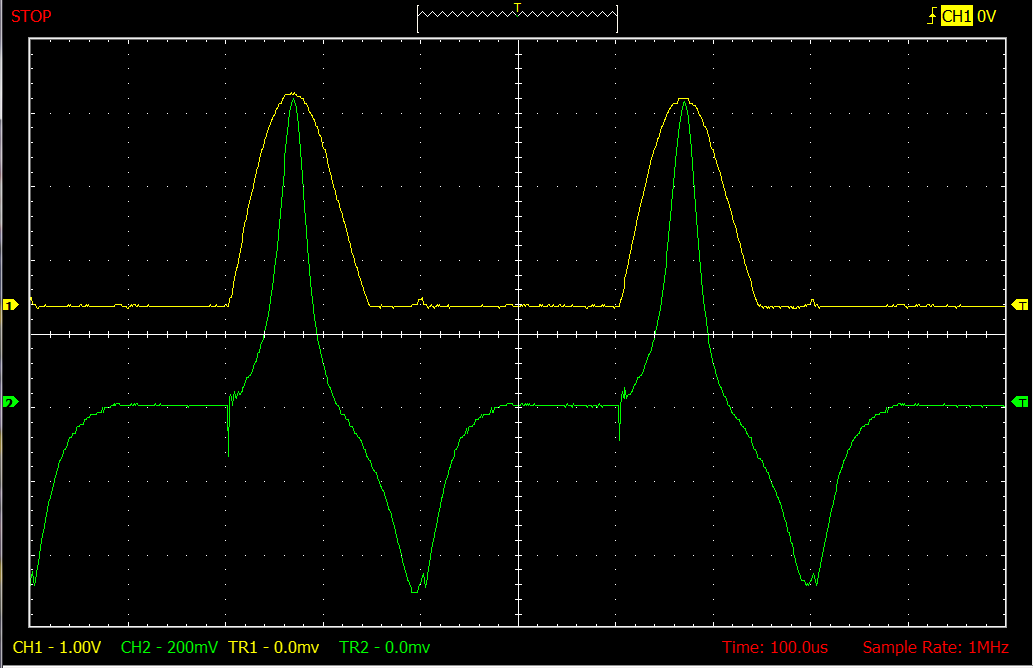Research website of Vyacheslav Gorchilin
2018-10-24
The resonance of the second kind
It's a completely different kind of resonance. It is not like the classic even in appearance, if you look at it through the screen of the oscilloscope (Fig. 2). Its resonance frequency depends not only on the inductance and capacitance, but also from the active resistance circuit, and the duty cycle. In this case, as in case of classical resonance, a decrease in a consumption current in a LCR circuit (Fig. 1). The mathematical dependence of these parameters among themselves is very different from the classical formula of Thomson . In this note we will propose its mathematical model and formulas to calculate and call him will further resonance of the second kind.
In the study of ferromagnets, some of the conditions for such a resonance has been found Dmitry S., D. Smith reflected them in their nomograms, and we will try to deduce an analytical model and formulas for its calculation.Fig.1. The equivalent circuit for the calculation of resonance of the second kindFig.2. The voltage waveform on the key SW1 (yellow beam) and the coil current L (green beam) at the resonance of the second kind
For this we need equivalenta diagram of process data taken from real coils and some assumptions. In the diagram (Fig. 1) submitted by: key SW1 that closes with a resonant frequency $$f_{r2}$$, where here and below under $$f_{r2}$$ we mean the resonance of the second kind (RVR); the resistance of the key is $$R_{S1}$$; the resonant circuit consisting of capacitance $$C$$, the inductance $$L$$ and resistance $$R_{L}$$; the power source E1 and the active resistance of connecting wires is $$R_{W}.$$ We believe that the power supply has a very small internal resistance. If not, then it can be added to $$R_{W}.$$
For further assumptions we will need the total resistance of the circuit, which is the summation of all its components: $R = R_{SW1} + R_{L} + R_{W} \qquad (1.1)$ it is Also assumed that the resistance of the key, wires and power source is much smaller than $$R_{L}$$.
For finding the resonant frequency of RVR were considered three possible mathematical model: $f_{r2} = {k_1 \over 2\pi\sqrt{L C} + k_2 L/R + k_3 R C } \qquad (1.2)$ $f_{r2} = {k_1 \over \sqrt{(2\pi\sqrt{L C})^2 + k_2 (L/R)^2 + k_3 (R C)^2 }} \qquad (1.3)$ $f_{r2} = k_1 \sqrt{f_r \frac{R}{L}}, \quad f_r = {1 \over 2\pi\sqrt{L C}} \qquad (1.4)$ where $$k_1, k_2, k_3$$ — constant coefficients, and $$f_r$$ is a classic resonance frequency according to the formula Thomson. These options were tested for the coils with different settings, with different materials of the conductor and core. Formulas (1.2) and (1.3) are very inaccurate for large relative values of capacitance and inductance, and (1.4) worked with an accuracy of 20% across the range. It took the basis with the coefficient $$k_1 = 1/2$$. It is interesting that this formula works for both conventional coils and bifilar windings burnout, almost non-inductance for both coils without core, and ferromagnetic and diamagnetic cores. So, to reflect this formula in its final form: $f_{r2} = \frac12 \sqrt{f_r \frac{R}{L}}, \quad f_r = {1 \over 2\pi\sqrt{L C}} \qquad (1.5)$

Conclusion using q-factor
A very interesting formula is obtained, if the output of the resonance of the second kind through the q-factor. It will show the nature of this resonance. For this formula you need to remember what the q-factor of oscillatory system at its operating frequency : $Q = \frac{\omega L}{R} \qquad (1.6)$ where: $$Q$$ is a factor, $$\omega$$ — circular frequency, which is thus: $$\omega = 2 \pi f_r$$. Substituting the expression (1.6) in (1.5), and performing the reduction that will get this formula: $f_{r2} = f_r \sqrt{\pi \over 2 Q} \qquad (1.7)$ from Here we immediately see the condition of equality of the classical resonant frequency and the resonant frequency of the second kind: $Q^{*} = \frac{\pi}{2} \qquad (1.8)$
The duty cycle
So far, we have investigated the resonance when the duty ratio of the key managers of pulses equal to 2 and the formula (1.5) is derived for this case. In contrast to classical resonance in RVR showed a strong dependence on this parameter. According to preliminary data, the dependence turns out like this: $f_{r2} = \frac{S}{4} \sqrt{\frac{S}{2} f_r \frac{R}{L}}, \quad f_r = {1 \over 2\pi\sqrt{L C}} \qquad (1.9)$ where: $$S$$ is the duty cycle.

Often confused with the duty cycle and the fill factor , so we again recall the difference between them. Under the ratio we mean the ratio of pulse period to pulse duration. The fill factor (duty cycle) is the reciprocal of the duty cycle: $$D = 1/S$$

The condition for the existence of resonance of the second kind
Waveforms for different types of winding are shown in figures 7 and 8 here. From them it becomes clear that the length of the pulse must be greater than the first (Romanovci) parts of it at least twice. Mathematically this can be described as: $T_I \ge 2 T_T, \quad T_I = 1/f_{r2}, \quad T_T = 2\pi\sqrt{L C} \qquad (1.10)$ substituting into this expression the known values of (1.5), and carrying with them the necessary reductions, we get the following relation: $\sqrt{\frac{L}{C}} \ge \frac{\pi}{2} R \qquad (1.11)$ But we know from the course of electrodynamics that the left part of the inequality is the impedance of the circuit, therefore a condition of obtaining RVR is reduced to a rather simple formula: $Z \ge \frac{\pi}{2} R, \quad Z = \sqrt{\frac{L}{C}} \qquad (1.12)$ where: $$Z$$ — characteristic impedance of the LC-circuit.
Above, we considered the condition when the duty ratio of 2. A more General formula, with any duty cycle and excreted by the substitution in inequality (1.10) equations (1.9): $Z \ge S^3 \frac{\pi}{16} R \qquad (1.13)$
It is a necessary but not a sufficient condition for the existence of resonance of the second kind. The author hopes that all terms are over time :)
Insights
The resonance of the second kind differs from the classical by the following features.
• It forms in the coil, the uneven distribution of the magnetic field at relatively low frequencies at which, in classical view, the inductance is considered to be a point and the field must be distributed evenly;
• A mathematical model of such a resonance is very different from Thomson formula, whereby the resonance frequency is inversely proportional to the square root of capacitance and inductance. In RVR, this frequency is inversely proportional to the fourth root from the container and root of 3/4 degree from the inductor;
• The classical resonance occurs, the phase shift between current and voltage 90 degrees. In RVR shift between current and voltage not;
• There are fundamental differences in the transmission power between the two coils when the classical resonance and RVR. The full report is here;
• In RVR the resonance frequency depends not only on the inductance and capacitance, but also from the active resistance of the circuit and the duty cycle;
• RVR requires certain conditions for its existence reflected in the formula (1.13).
The materials used
1. Wikipedia. The Formula Of Thomson.
2. Wikipedia. The q-factor.
3. Wikipedia. Duty cycle.